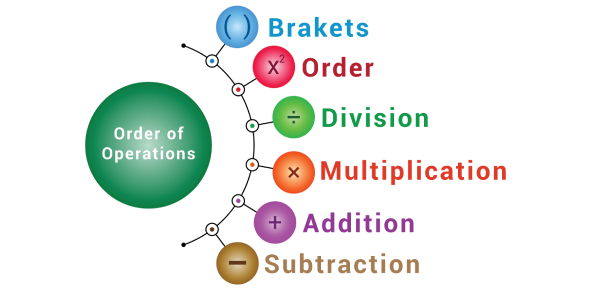# Bodmas Quiz Questions And Answers

10 Questions | Attempts: 5085
ShareSettingsHow strong is your basic math? Are all your concepts clear in this subject? Play this BODMAS quiz, specially created for math students, and solve all these problems given here. BODMAS is an acronym used to solve different sets of operations in calculations. It stands for Brackets, Order, Division, Multiplication, Addition, and Subtraction. All mathematical calculations are solved in this order only. So, let's see how well you perform on this quiz.

• 1.
Solve this equation: 30 + 12 - 8 =
• A.

58

• B.

34

• C.

12

• D.

43

• 2.
Solve this equation:35/7 + 3 =
• A.

3.5

• B.

7

• C.

8

• D.

16.5

• 3.
Solve this equation:2 + 3 + 7 * (16/8) =
• A.

19

• B.

22

• C.

20

• D.

18

• 4.
Solve this equation:5 * 2 + (11 + 8) - 18/9 =
• A.

28

• B.

27

• C.

26

• D.

25

• 5.
Solve this equation:(11 - 8) * 3 + 7 + 27 - 3 =
• A.

50

• B.

41

• C.

30

• D.

40

• 6.
Solve this equation:(12/3) + 3 =
• A.

7

• B.

8

• C.

9

• D.

10

• 7.
Solve this equation:10 * 8 + 2 =
• A.

66

• B.

45

• C.

54

• D.

82

• 8.
Solve this equation:6/3 + 24 - 25/5 =
• A.

22

• B.

21

• C.

23

• D.

20

• 9.
Solve this equation:4 + 2 * 18/6 - 9 =
• A.

2

• B.

-1

• C.

1

• D.

-2

• 10.
Solve this equation:   8 * 3 + 40/5 - 8 =
• A.

25

• B.

-24

• C.

-25

• D.

24

## Related TopicsBack to top
×

Wait!
Here's an interesting quiz for you.